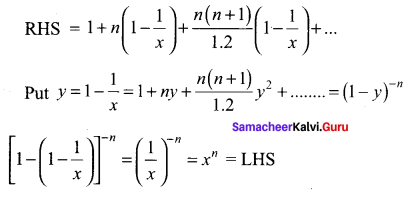## Tamilnadu Samacheer Kalvi 11th Maths Solutions Chapter 5 Binomial Theorem, Sequences and Series Ex 5.4

Question 1.
Expand the following in ascending powers of x and find the condition on x for which the binomial expansion is valid.
Solution: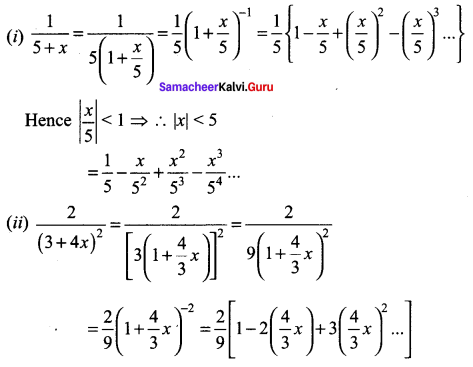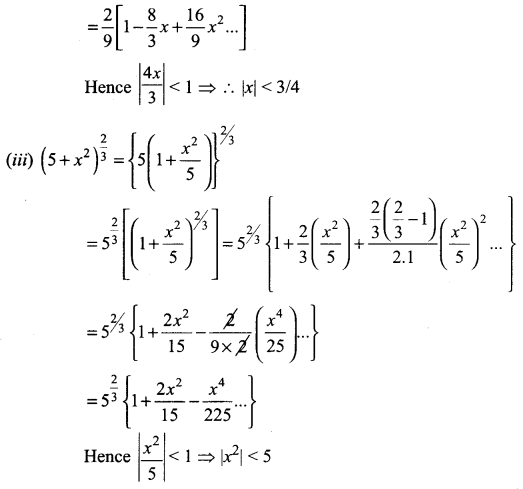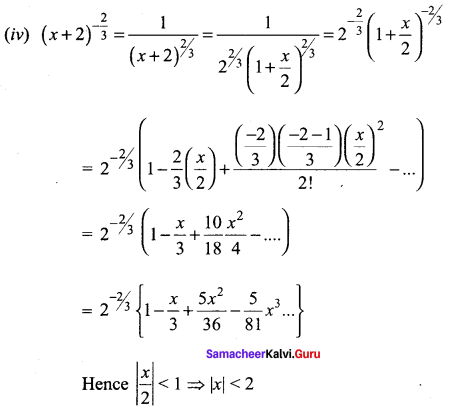Question 2.
Find $$\sqrt{1001}$$ approximately (two decimal places).
Solution: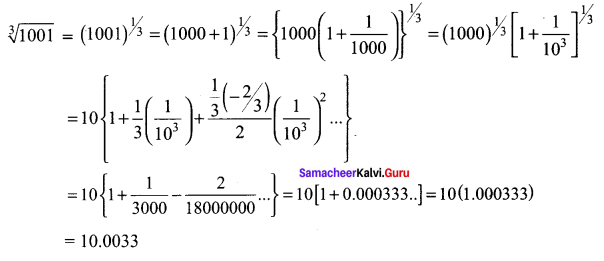Question 3.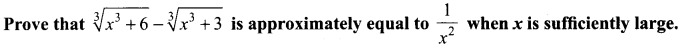Solution: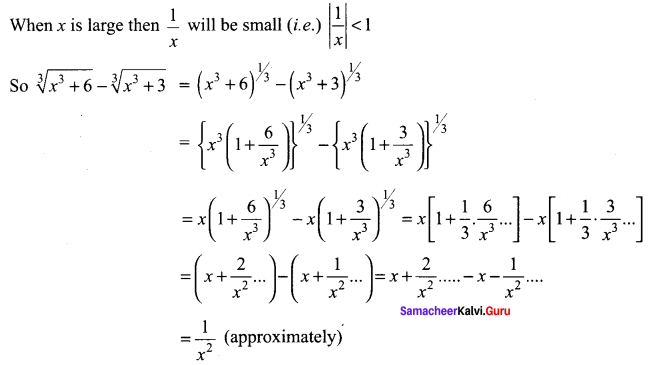Question 4.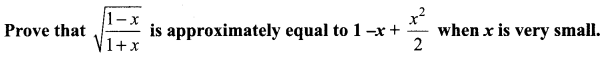Solution: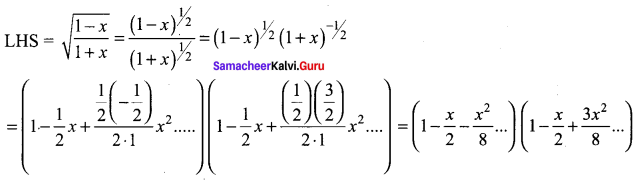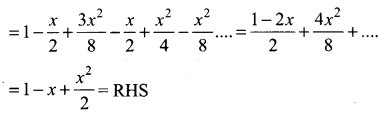Question 5.
Write the first 6 terms of the exponential series
(i) e5x
(ii) e-2x
(iii) $$e^{\frac{x}{2}}$$
Solution: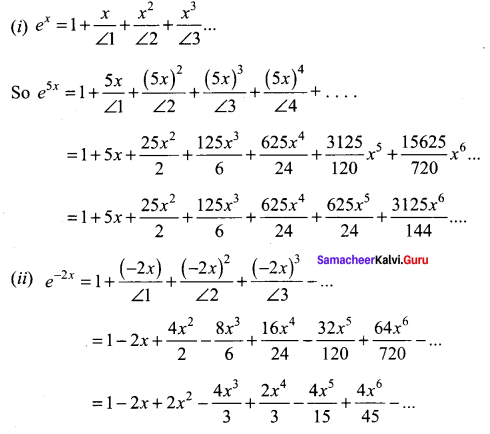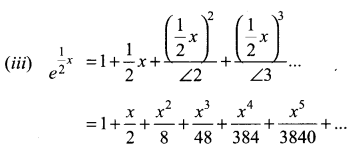Question 6.
Write the first 4 terms of the logarithmic series
(i) log(1 + 4x),
(ii) log(1 – 2x),
(iii) $$\log \left(\frac{1+3 x}{1-3 x}\right)$$
(iv) $$\log \left(\frac{1-2 x}{1+2 x}\right)$$.
Find the intervals on which the expansions are valid.
Solution: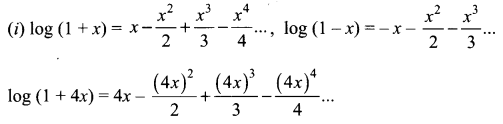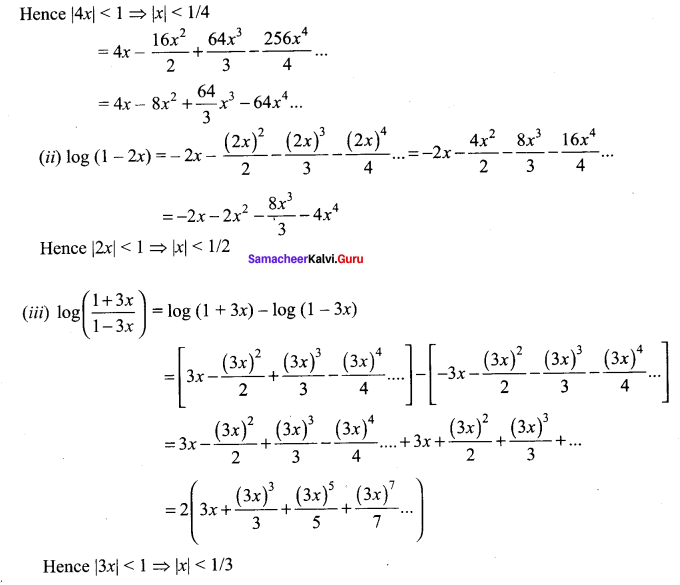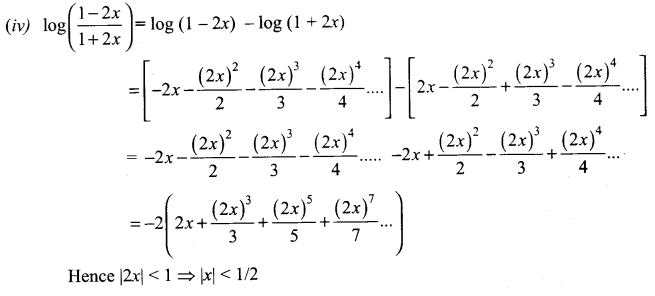Question 7.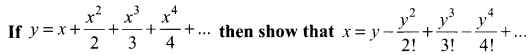Solution: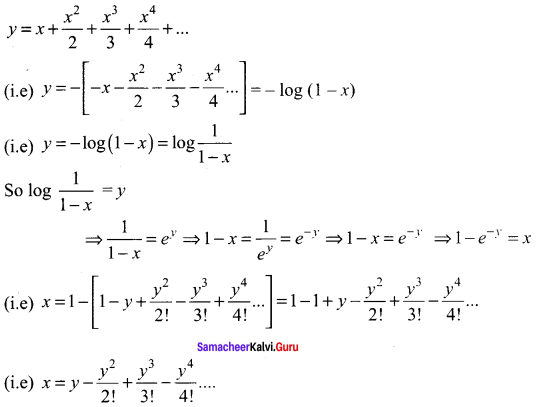Question 8.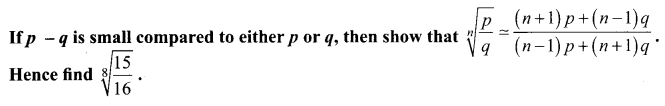Solution: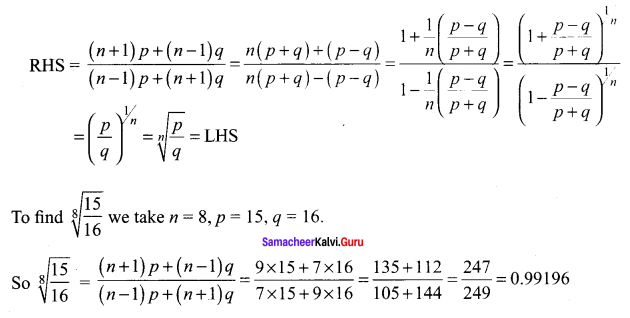Question 9.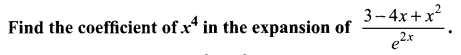Solution: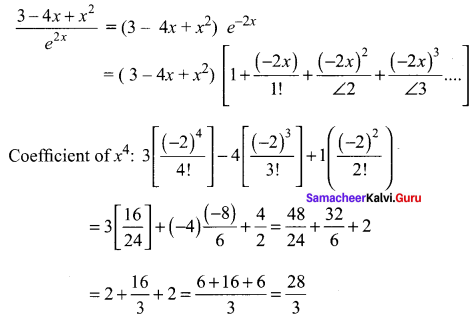Question 10.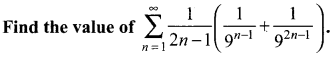Solution: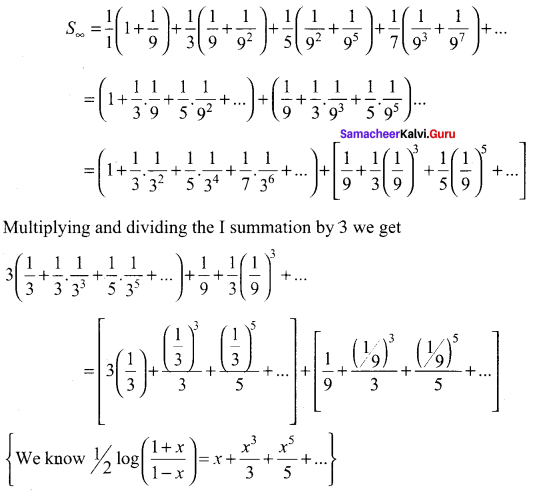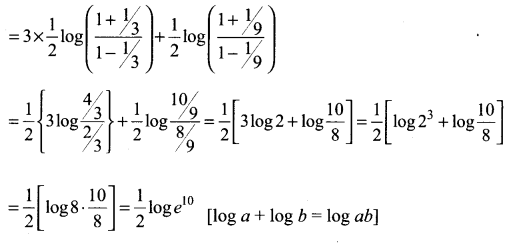### Samacheer Kalvi 11th Maths Solutions Chapter 5 Binomial Theorem, Sequences and Series Ex 5.4 Additional Questions

Question 1.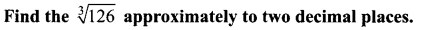Solution: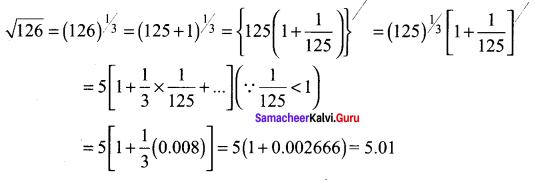Question 2.
Write the four terms in the expansions of the following: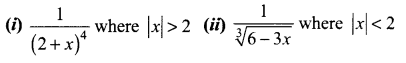Solution: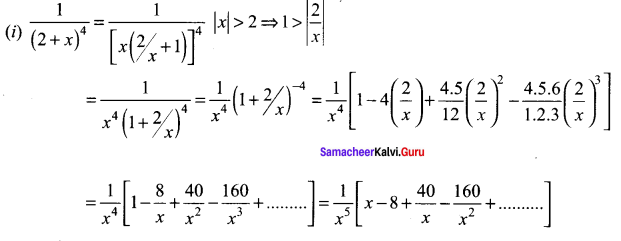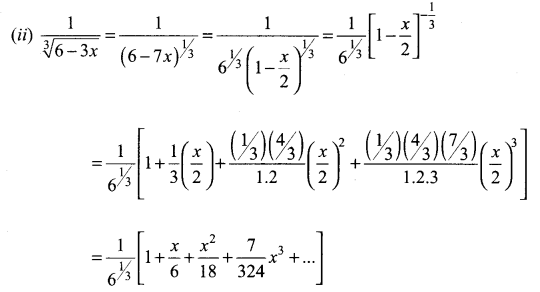Question 3.
Evaluate the following: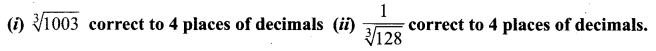Solution: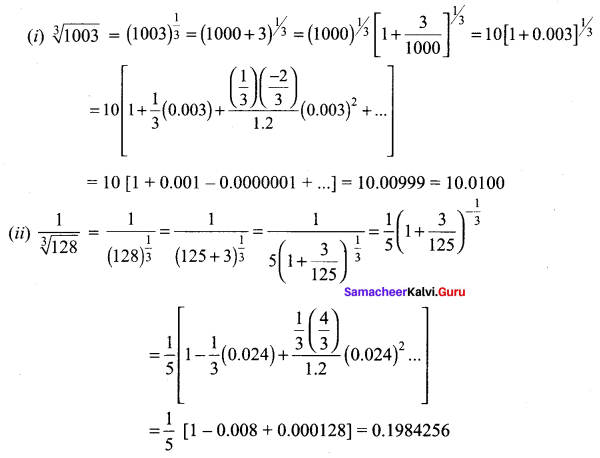Question 4.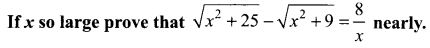Solution: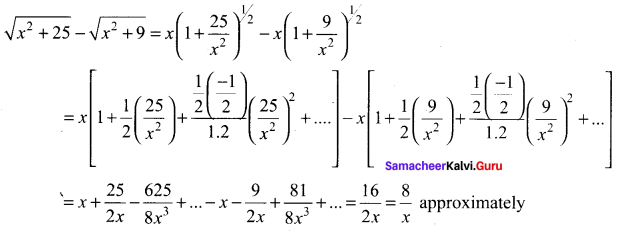Question 5.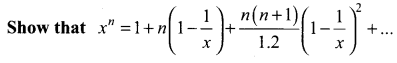Solution: